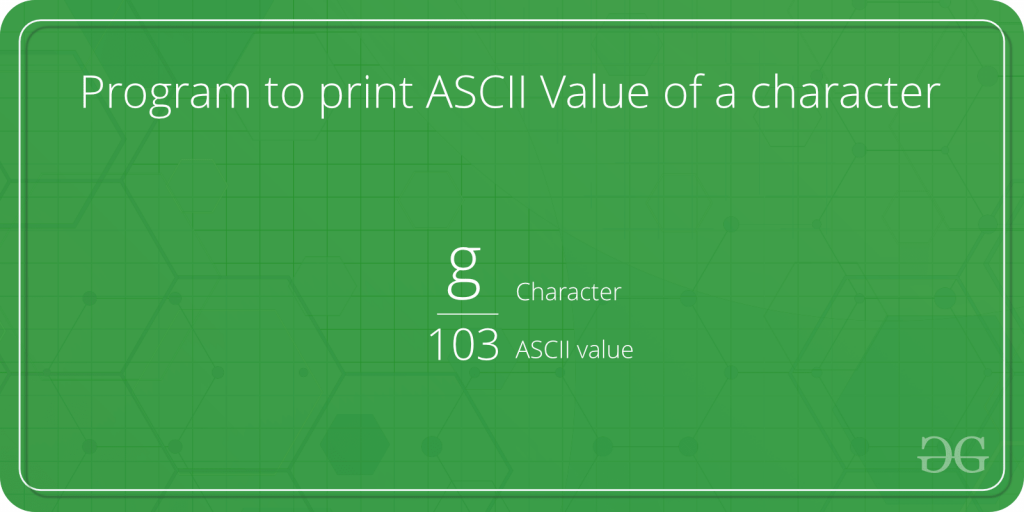GFG App
Open AppBrowser
Continue

# Program to print ASCII Value of a character

Given a character, we need to print its ASCII value in C/C++/Java/Python.Examples :

Input :
Output : 97

Input : D
Output : 68

Here are few methods in different programming languages to print ASCII value of a given character :

1. Python code using ord function : ord() : It converts the given string of length one, returns an integer representing the Unicode code point of the character. For example, ord(‘a’) returns the integer 97.

## Python

 `# Python program to print  ` `# ASCII Value of Character ` ` `  `# In c we can assign different ` `# characters of which we want ASCII value  ` ` `  `c ``=` `'g'` `# print the ASCII value of assigned character in c ` `print``(``"The ASCII value of '"` `+` `c ``+` `"' is"``, ``ord``(c)) `

Output

`("The ASCII value of 'g' is", 103)`

Time Complexity: O(1)
Auxiliary Space: O(1)

1. C code: We use format specifier here to give numeric value of character. Here %d is used to convert character to its ASCII value.

## C

 `// C program to print ` `// ASCII Value of Character ` `#include ` `int` `main() ` `{ ` `    ``char` `c = ``'k'``; ` ` `  `    ``// %d displays the integer value of a character ` `    ``// %c displays the actual character ` `    ``printf``(``"The ASCII value of %c is %d"``, c, c); ` `    ``return` `0; ` `} `

Output

`The ASCII value of k is 107`

Time Complexity: O(1)
Auxiliary Space: O(1)

1. C++ code: Here int() is used to convert a character to its ASCII value.

## CPP

 `// CPP program to print ` `// ASCII Value of Character ` `#include ` `using` `namespace` `std; ` `int` `main() ` `{ ` `    ``char` `c = ``'A'``; ` `    ``cout << ``"The ASCII value of "` `<< c << ``" is "` `<< ``int``(c); ` `    ``return` `0; ` `} `

Output

`The ASCII value of A is 65`

Time Complexity: O(1)
Auxiliary Space: O(1)

1. Java code : Here, to find the ASCII value of c, we just assign c to an int variable ascii. Internally, Java converts the character value to an ASCII value.

## Java

 `// Java program to print ` `// ASCII Value of Character ` `public` `class` `AsciiValue { ` ` `  `    ``public` `static` `void` `main(String[] args) ` `    ``{ ` ` `  `        ``char` `c = ``'e'``; ` `        ``int` `ascii = c; ` `        ``System.out.println(``"The ASCII value of "` `+ c + ``" is: "` `+ ascii); ` `    ``} ` `} `

Output

`The ASCII value of e is: 101`

Time Complexity: O(1) // since no loop is used the algorithm takes up constant time to perform the operations
Auxiliary Space: O(1) // since no extra array is used so the space taken by the algorithm is constant

1. C# code : Here, to find the ASCII value of c, we just assign c to an int variable ascii. Internally, C# converts the character value to an ASCII value.

## CSHARP

 `// C# program to print ` `// ASCII Value of Character ` `using` `System; ` ` `  `public` `class` `AsciiValue  ` `{ ` `    ``public` `static` `void` `Main() ` `    ``{ ` ` `  `        ``char` `c = ``'e'``; ` `        ``int` `ascii = c; ` `        ``Console.Write(``"The ASCII value of "` `+  ` `                        ``c + ``" is: "` `+ ascii); ` `    ``} ` `} ` ` `  `// This code is contributed  ` `// by nitin mittal `

Output

`The ASCII value of e is: 101`

Time Complexity: O(1)
Auxiliary Space: O(1)

1. JavaScript code: Here, to find the ASCII value of the character, the charCodeAt() method is used to get the ASCII value of the character at a specific index in a string.

## Javascript

 `// JavaScript program to print ` `// ASCII Value of Character ` ` `  `console.log(``"The ASCII value of "` `+ ``'A'` `+ ``" is "` `+ ``'A'``.charCodeAt(0)); ` ` `  `// This Code is Contributed by Prasad Kandekar(prasad264)`

Output:

`The ASCII value of A is 65`

Time Complexity: O(1)
Auxiliary Space: O(1)

Here is a method to print the ASCII value of the characters in a string using python:

## Python3

 `print``(``"Enter a String: "``, end``=``"") ` `text ``=` `input``() ` `textlength ``=` `len``(text) ` `for` `char ``in` `text: ` `    ``ascii ``=` `ord``(char) ` `    ``print``(char, ``"\t"``, ascii) `

Input:

`Enter a String: Aditya Trivedi`

Output:

```A 65
d 100
i 105
t 116
y 121
a 97
32
T 84
r 114
i 105
v 118
e 101
d 100
i 105```

Time Complexity: O(N), where N is the length of the input string.
Auxiliary Space: O(1)

My Personal Notes arrow_drop_up